Math Practice: Working with Radical Expressions

What are radical expressions? Before you start thinking about political revolutions, you can rest assured that I don’t mean that kind of radical expression! I’m talking about radicals in mathematics. You know, square roots, cube roots, and the like. Simplifying radicals and working with radical expressions are important skills in Algebra.“Oh sorry. We were talking about math??? . . . RETREAT!”

Image By Everett Historical

So what exactly are radical expressions? Basically any mathematical expression that involves a radical. Radical comes from the Latin radix, which means root. So we think of square roots, cube roots, and even 100th roots of numbers.

An nth root of a number x is a number y such that the nth power of y gives you back x. Square root is just another name for the 2nd root. Does it sound confusing? Don’t worry, we’ll see how to use this definition in the examples below.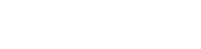So for example, √9 = 3, because 32 = 9. And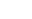= 2, because 25 = 32.

See how radicals are like the opposite of powers? (Technically, we say that finding the nth root is the inverse operation to finding the nth power.)

Radical expressions may include variables or only numbers. The expression within the radical is called the radicand. The tiny number in the upper left of the radical symbol is the index. Square roots have index 2, implied but not written in the radical notation.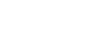A radical expression consists of the radical symbol, the radicand (in green), and the index (in red).

Radical expressions are equivalent to expressions with fractional powers. The rule is pretty simple: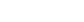Rule for fractional exponents

In other words, any time you see an exponent like 1/n, then you can regard that as an nth root, and vice versa.

This is especially important when variables are involved, because it shows how roots essentially turn into division of exponents by the index.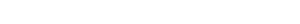For more about this point, as well as a list of practice problems, go check out Math Practice: Negative and Fractional Exponents.

Sometimes radical expressions can be simplified. The simplest case is when the radicand is a perfect power, meaning that it’s equal to the nth power of a whole number.

For example the perfect squares are: 1, 4, 9, 16, 25, 36, etc., because 1 = 12, 4 = 22, 9 = 32, 16 = 42, 25 = 52, 36 = 62, and so on. Therefore, we have √1 = 1, √4 = 2, √9 = 3, etc.

Perfect cubes include: 1, 8, 27, 64, etc. So,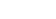, and so on.

Variables with exponents also count as perfect powers if the exponent is a multiple of the index. For instance, x2 is a perfect square. But so are y4 and w18.

Working with Perfect Powers

The key concept is that an nth root of a perfect nth power will completely simplify to a non-radical expression.

For example,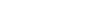.

The square root of 81 is 9, while the square root of x6 is (x6)1/2 = x3.

Pulling Out Perfect Power Factors

If the radicand is not a perfect power, then you still might be able to factor it into part that is a perfect power. Then you can simplify that part of it and leave the rest within the radical.

Let me show you what I mean.

Suppose you want to take the cube root of 24x4. Well 24 is not a perfect cube… but one of its factors, 8, definitely is! Similarly, x4 is not a perfect cube, but we can factor it as x3·x, and x3 is a perfect cube.Notice how the cube root of 8x3 simplifies to 2x, which we then write outside of the radical expression (in green). The leftover 3x cannot simplify and must remain within the radical.

Rationalizing the Denominator

Finally, we have to discuss another method of simplifying radicals called rationalizing the denominator. This is a technique for rewriting a radical expression in which the radical shows up on the bottom of a fraction (denominator).

There are two basic cases to worry about. Also, for pretty much every problem in your Algebra class, you only have to do this for square roots. (Rationalizing roots with higher index can get a lot more complicated.)

• The denominator is a single term involving a radical. In this case, multiply both top and bottom of the fraction by the radical expression.
• The demoninator has two terms, like a + √b. Here, you must multiply both the top and bottom of the fraction by the conjugate, a – √b. And if you had a – √b to begin with, its conjugate would be a + √b. (Just flip the sign of the radical.)

Here’s an example showing the technique in practice.Words to the Wise — Be Careful in Simplifying Radicals

You may have noticed that we sometimes were able to simplify part of the radical expression by pulling out a perfect power.

Be Careful! This technique only works because we are dealing with factors, rather than terms. Factors are things that are multiplied together. Terms are things that are added or subtracted.

So you may do this: √36 · x = 6√x.

But you MAY NOT do this: √36 + x = 6 + √x   (Big No-no).

In other words, radical expressions do not break apart over plus (+) or minus (–) signs.

Even the best students make this mistake from time to time. So just be aware and try to catch it early before it becomes a habit.

Practice Problems

1. 4√24 – 7√150

2.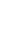3.

4.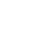5.

6.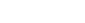Solutions

1. Pull out the perfect squares.

4√4 · 6 – 7√25 · 6 = 4(2√6) – 7(5√6) = 8√6 – 35√6 = -27√6

2. Multiply both the top and bottom by the radical.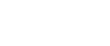3.

4. Don’t forget to multiply both the top and bottom of the fraction by the conjugate!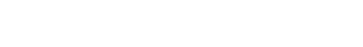5.

6. Pull out perfect square factors.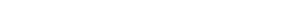Author

•Shaun earned his Ph. D. in mathematics from The Ohio State University in 2008 (Go Bucks!!). He received his BA in Mathematics with a minor in computer science from Oberlin College in 2002. In addition, Shaun earned a B. Mus. from the Oberlin Conservatory in the same year, with a major in music composition. Shaun still loves music -- almost as much as math! -- and he (thinks he) can play piano, guitar, and bass. Shaun has taught and tutored students in mathematics for about a decade, and hopes his experience can help you to succeed!